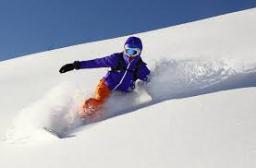# Remaining 4448

3/4 of the class went skiing. Of those who stayed home, 1/3 fell ill, and the remaining six were at a math camp. How many students does the class have?

x =  36

### Step-by-step explanation:

3/4 x + (1-3/4) x/3 + 6 = x

3/4·x + (1-3/4)·x/3 + 6 = x

2x = 72

x = 72/2 = 36

x = 36

Our simple equation calculator calculates it.Did you find an error or inaccuracy? Feel free to write us. Thank you!

Tips for related online calculators
Need help calculating sum, simplifying, or multiplying fractions? Try our fraction calculator.
Do you have a linear equation or system of equations and looking for its solution? Or do you have a quadratic equation?